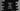# Python program to add items to a list without using append()## How to add items to a python list without using append():

We can add items to a python list without using append. There are many ways to do that. In this post, we will learn a few different ways to add items to a list with examples.

### Method 1: By using ’+‘:

This is the easiest way to add items to a list in python. Plus operator or + can be used to add items to a list. We can add another list to a given list. Let’s take a look at the below program:

``````given_list = [1, 2, 3, 4, 5]
new_list = given_list + [6, 7, 8]

print(new_list)``````

Here, We are creating new_list by adding items to the given_list. It will print the below output:

``[1, 2, 3, 4, 5, 6, 7, 8]``

### Method 2: Using extend:

extend() is a method defined in python list which can be used to append a list to the end of a list. extend method modifies the original caller list and it returns None. Let’s take a look at the example below:

``````given_list = [1, 2, 3, 4, 5]
given_list.extend([6, 7, 8])

print(given_list)``````

It will print the below output:

``[1, 2, 3, 4, 5, 6, 7, 8]``

### Method 3: Using slicing:

slicing is another way to add one or more items to a list. For example, the below program adds the items to the start of the given list:

``````given_list = [1, 2, 3, 4, 5]
given_list[:0] = [6, 7, 8]

print(given_list)``````

It will print the below output:

``[6, 7, 8, 1, 2, 3, 4, 5]``

Similarly, the below script adds items to the end of the list:

``````given_list = [1, 2, 3, 4, 5]
size = len(given_list)

given_list[size:] = [6, 7, 8]

print(given_list)``````

It will print:

``[1, 2, 3, 4, 5, 6, 7, 8]``